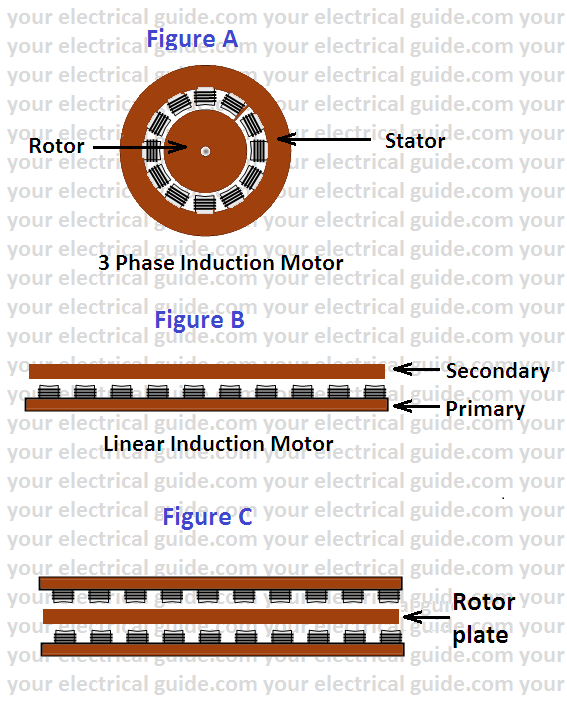# Linear Induction Motor Working Principle

Hi friends, in this article, I am going to describe the linear induction motor working principle. This article will provide you answers of various questions about the LIM.

A linear induction motor is a developed version of cylindrical induction motor. It is special type of induction motor which gives linear motion instead of rotational motion as in the case of a conventional induction motor.

If the induction motor of Figure (A) is cut axially and spread out flat as shown in Figure(B), it corresponds to a linear induction motor (LIM).

The secondary conductor now appears in form of a sheet. It is normally a conducting plate made of either copper or aluminum in which interaction currents are induced.

Either the primary or secondary could be made mobile. The stationary member must, of course, be continuous throughout the length of intended travel.Magnetic attraction between members can be balanced out in a rotary machine but can’t be balanced out in a flat machine easily. To balance out the magnetic attraction, the primary is placed on the both sides of the secondary (Figure C). This construction is commonly adopted for traction.

# Linear Induction Motor Working Principle

The primary has a 3-phase distributed winding placed in slots. It operates on the same principle on which a cylindrical induction motor operates.

When the phase primary of a LIM is energized from a three-phase source, magnetic field moving in a straight line from one end to the other end at a linear synchronous speed Vs is developed.

The linear synchronous speed is given as:

Vs = 2tf meters/second

Where t = pole pitch in meters
f = supply frequency.

Here, it is to be noted that the synchronous speed is not governed by the number of poles and therefore any desired linear speed can be achieved by adjusting pole pitch.

As flux moves linearly, it induces currents in the rotor plate. The induced currents interacting with the field produce thrust on rotor plate and drags the rotor plate along within the same direction. This is the basic “linear induction motor working principle”.

Like cylindrical induction motor, LIM also runs at speed less than synchronous speed. It can’t run at synchronous speed.  Reasons are the same that are in the case of a cylindrical induction motor.

Slip of linear induction motor, s = (vs – v)/vs

Tractive effort or thrust, F can be controlled by varying both frequency and voltage so that induction density remains constant.

## Transverse Edge Effect and End Effect

There are two particular effects which occur in LIM but not in a cylindrical induction motor. These effects are transverse edge effect and end effect.

The path of induced current in the secondary is not well defined because secondary of LIM is a solid conducting plate. The portion of current paths parallel to the direction of motion of secondary does not produce any useful thrust but only contribute towards losses.

This is known as the transverse edge effect in linear induction motor because the current paths parallel to the direction of motion are more at the edges of conducting plate.

In a LIM with short primary, the current paths towards the end of field structure on the conducting plate go beyond the field structure and such portions of current paths do not produce any useful thrust but only contribute toward motor losses. This is known as end effect in the linear induction motor. It can be effectively reduced by increasing the number of poles on the motor.

## Applications of Linear Induction Motor

• The linear induction motor is commonly used for traction wherein the finite length primaries carried out on the vehicle while the secondary takes the form of a continuous conducting rail (aluminum is used).
• LIM has superiority over cylindrical induction motor for speeds over 200 kmph. It provides an excellent source of motive power for magnetically suspended trains where cylindrical induction motor fails.
•

• It is used in automatic sliding doors.
• It can be used in conveyors, traveling cranes, haulers etc.
• The use of LIM is limited to only a few applications till now due to design difficulties and economic considerations.

## Advantages and Disadvantages of Linear Induction Motor

The advantages of linear induction motors are:

• Low initial cost.
• Low maintenance cost due to the absence of rotating parts.
• No limitation of maximum speed due to centrifugal forces.
• No overheating of the rotor.
• Batter power to weight ratio.

The disadvantages of linear induction motors are:

• A typical air gap in LIM is of the order of 25 mm while in a cylindrical motor it is about 1 mm i.e. it has a larger air gap which results in lower power factor.
• LIM has a much higher rotor resistance, operates at high slip at given thrust and has correspondingly low efficiency.
• Very high capital cost of reaction rail fixed along the center line of the track.

Thanks for reading about linear induction motor working principle.

## Three Phase Induction Motor | All Posts

© www.yourelectricalguide.com/ linear induction motor working principle.